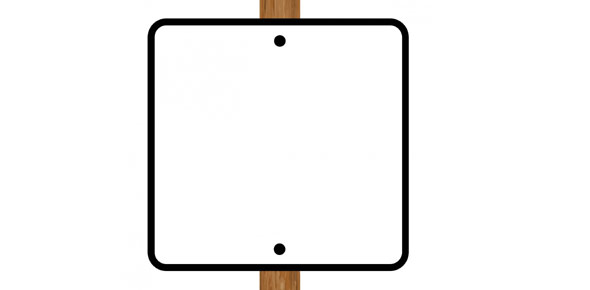# Quiz On Completing The Square

5 Questions | Attempts: 994SettingsThis quiz covers all areas of completing the square

• 1.
What is the first thing that should be done to complete the square on 4x^2+8x+16=0
• A.

Subtract 16 from both sides

• B.

Divide 8 by 2 and square it

• C.

Divide by 4

• D.

Factor

• 2.
The goal of completing the square is to create a
• 3.
X^2-8x+16 would factor into...
• A.

(x-4)^2

• B.

(x+4)^2

• C.

(x+8)^2

• D.

(x-8)^2

• 4.
What term would make x^2+6x a perfect square trinomial? (you need to include the sign)
• 5.
What would need to be done next in order to complete the square for x^2-8x    =10?
• A.

Move 10

• B.

Factor

• C.

Take half of 8 and square it.

• D.

Factor out an x

## Related TopicsBack to top
×

Wait!
Here's an interesting quiz for you.